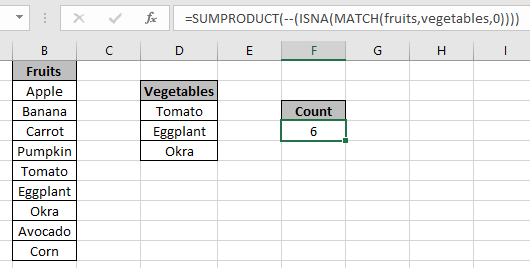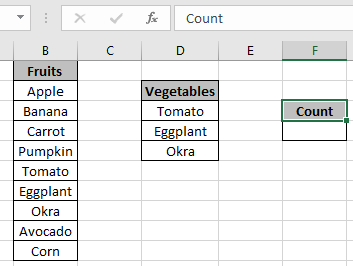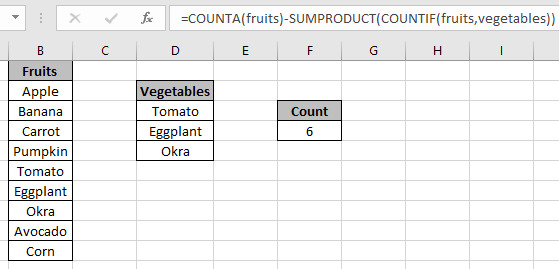# Count cells which are not equal to many things in excel

In this article, we will learn about how to Count cells which are not equal to many things in Excel.

In simple words, consider a scenario in which we are required to count the data based on specific criteria in an array. counting cells that do not contain values to catch cell value with excel function explained here with an example. Use Wildcards characters for catching strings and perform functions on it.

For this we will be using the following functions.

Now we will get to learn about them in brief. We have different methods to do the same. So we will see it one by one.
First method

Count cell that do not contain values in a range. Here we have a formula to do so. We will use the MATCH function to get where are the values which needed to be counted and ISNA function catches #NA error. Then SUMPRODUCT returns the sum of the values.

Generic formula:

 = SUMPRODUCT ( -- ( ISNA ( MATCH ( range, values, 0)))

Variables:

range: array of cell to count
values: array of values to ignore in range.
Example
Let’s understand this function using it in an example.
Here we have fruits in one column and vegetables in another. The first lust have some vegetables in the list and we need to count only the fruits not considering vegetables.Use the formula:

 = SUMPRODUCT ( -- ( ISNA ( MATCH (fruits , vegetables , 0 ) ) ) )

fruits: named range for the array B2:B10
Vegetables: named range used for the array D3:D5
Explanation:

• MATCH function returns the position of each index where values are matched in range or returns #NA error where not found.
• ISNA function catches #NA error and returns TRUE for the #NA error and FALSE elsewhere.
• -- operator converts all TRUEs to 1s and FALSEs to 0s.
• SUMPRODUCT function returns the sum of all the numbers in the array which indeed is the count of cells where values are not considered.As you can see the formula returns the count of fruits not considering any value from vegetables.
Second method:
We will be considering other methods because different types of problems have different solutions. Use of the COUNTIFS function in Excel.
Generic formula:

 = COUNTIFS ( range, "<>value1", range, "<>value2", …)

Variables:
range: array of cell to count
value1: value to ignored in range
value2: value to ignored in range
Example
Let’s understand this function using it in an example.
Here we have fruits in one column and vegetables in another. The first column has some vegetables in the list and we need to count only the fruits not considering vegetables.Use the formula:

 = COUNTIFS ( fruits , "<>" & D3, fruits , "<>" & D4 , fruits , "<>" & D5 )

fruits: named range for the array B2:B10

D3 , D4 & D5 are the cell references used in the formula

Explanation:

• Here the range is checked with the individual values and returns the count of cells.
• <> operator is not equals to
• & operator, concatenate values with cell reference value.

Use the formula:

 = COUNTIFS ( fruits , "<>" & D3, fruits , "<>" & D4 , fruits , "<>" & D5 )

fruits: named range for the array B2:B10

D3,D4 &D5 are the cell references used in the formula
Explanation:

• Here the range is checked with the individual values and returns the count of cells.
• <> operator is not equals to
• & concatenate the operator with value used by cell reference.As you can see the formula returns the count of fruits not considering any value from vegetables.
Third method
We will be considering other methods because different types of problems have different solutions. Use of the COUNTA function in Excel. Here the logic is calculate the count of cells having values and then subtract it from the count of all the values in range.

Generic formula:

 = COUNTA (range) - SUMPRODUCT ( COUNTIF ( range, values )

Variables:
range: array of cell to count
values: array of values to ignore in range.
Example
Let’s understand this function using it in an example.
Here we have fruits in one column and vegetables in another. The first lust have some vegetables in the list and we need to count only the fruits not considering vegetables.Use the formula:

 = COUNTA (fruits) - SUMPRODUCT ( COUNTIF ( fruits , vegetables ) )

fruits: named range for the array B2:B10
Vegetables: named range used for the array D3:D5
Explanation:

• COUNTIF function counts the cells in fruits having vegetables.
• SUMPRODUCT gets the sum considering all the values.
• COUNTA function calculates the count of non empty cells in a range.
• The difference will get the count of cells (fruits) not having values (vegetables).As you can see the formula returns the count of fruits not considering any value from vegetables.
Hope you understood how to Count cells which are not equal to many things in Excel. Explore more articles on Excel Count functions here. Please feel free to state your query or feedback for the above article.

Related Articles

COUNTIFS with Dynamic Criteria Range: Count cells having dynamic criteria range using COUNTIFS function.

COUNTIFS Two Criteria Match: Count cells having multiple criteria range using COUNTIFS function.

COUNTIFS With OR For Multiple Criteria: Count cells using the logic functions with COUNTIFS function.

The COUNTIFS Function in Excel: use of COUNTIFS function to count cells having multiple criteria

How to Use Countif in VBA in Microsoft Excel: Use the COUNTIF function in VBA to count cells.

How to use wildcards in excel: Catch phrases and keywords using the explained wildcard characters in excel

Popular Articles

50 Excel Shortcut to Increase Your Productivity

Edit a dropdown list

If with conditional formatting

If with wildcards

Vlookup by date

Terms and Conditions of use

The applications/code on this site are distributed as is and without warranties or liability. In no event shall the owner of the copyrights, or the authors of the applications/code be liable for any loss of profit, any problems or any damage resulting from the use or evaluation of the applications/code.